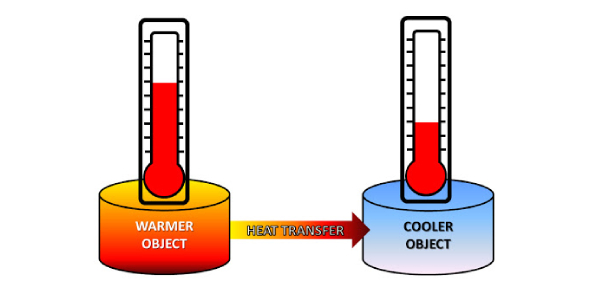# Thermal Engineering Quiz: What Do You Know About Heat Transfer?

9 Questions | Total Attempts: 4953SettingsWhat do you know about heat transfer? Convection, conduction, and thermal radiation; these are the core three types of heat transfer that you’ll come up against in the following thermal engineering quiz as we look at how heat can be transferred from one place to another. What can you tell us about heat transfer and some of the examples of how it takes place? Take the quiz, and we’ll find out!

• 1.
WHAT IS AN EXAMPLE OF RADIATION?
• A.

A CAMP FIRE

• B.

HOT AIR BALLOON

• C.

WIND CURRENT

• D.

COPPER

• 2.
THE LIGHT BULB IS AN EXAMPLE OF -
• A.

CONDUCTION

• B.

• C.

ELECTROMAGNETIC

• D.

CONVENTION

• 3.
DEFINE CONDUCTION?
• A.

THE UP AND DOWN MOVEMENT OF GASES- LIQUID TRANSFERS TO HAET.

• B.

THE PRIMARY METHOD BY WHIC HEAT MOVES GASES THROUGH LIQUID.

• C.

HEAT TRANSFER TO AND THROUGH SOME MATERIALS.

• D.

TRANSFER OF HEAT BETWEEN SUBSTANCES THAT ARE IN DIRECT CONTACT WITH EACH OTHER .

• 4.
WHEN ELECTROMAGENTIC WAVES TRAVEL THROUGH THE SPACE WHAT THEY CALLED?
• A.

ELECTROMAGNETIC

• B.

CONVENTION

• C.

• D.

CONDUCTION

• 5.
WHERE DO ELECTROMAGNETIC WAVES TRAVEL THROUGHT?
• A.

EVERYWHERE

• B.

ALL THE SPACE

• C.

EMPTY SPACE

• D.

ALL THE ABOVE

• 6.
GIVE AN EXAMPLE OF CONVECTION?
• A.

A LIGHT BULB

• B.

HOT AIR BALLOON

• C.

A CAMP FIRE

• D.

A MICROWAVE OVEN

• 7.
CONVENTION IS THE UP AND DOWN MOVEMENT OF GASES AND LIQIDS CAUSE BY -
• A.

HEAT TRANSFER

• B.

ELECTROMAGNETIC

• C.

TRANSFER THE HEAT TO THE OBJECT

• 8.
WHEN THE ELECTROMAGNETIC WAVES CONECT WITH THE OBJECT THE WAVES TRANSFER TO WHAT-
• A.

OBJECT

• B.

SPACE

• C.

WAVES

• D.

HEAT

• 9.
WHAT KIND OF METHOD DOES COVENTION IS?
• A.

SECONDARY METHOD

• B.

PRIMARY METHOD

• C.

ALL THE ABOVE

Related TopicsBack to top